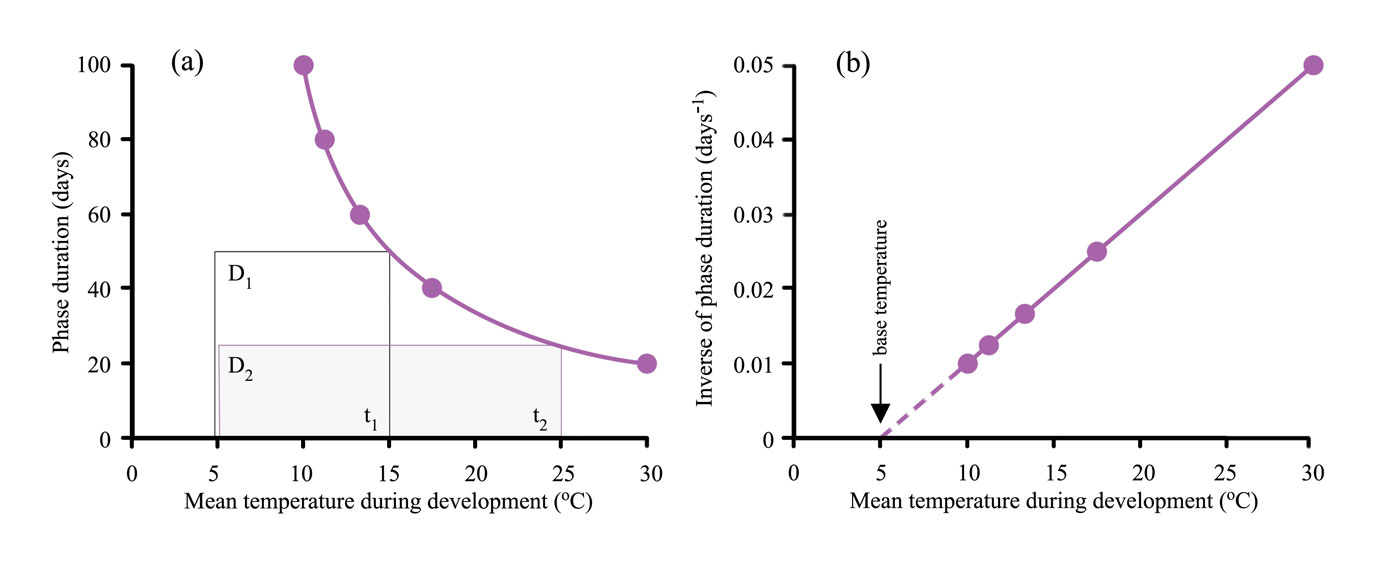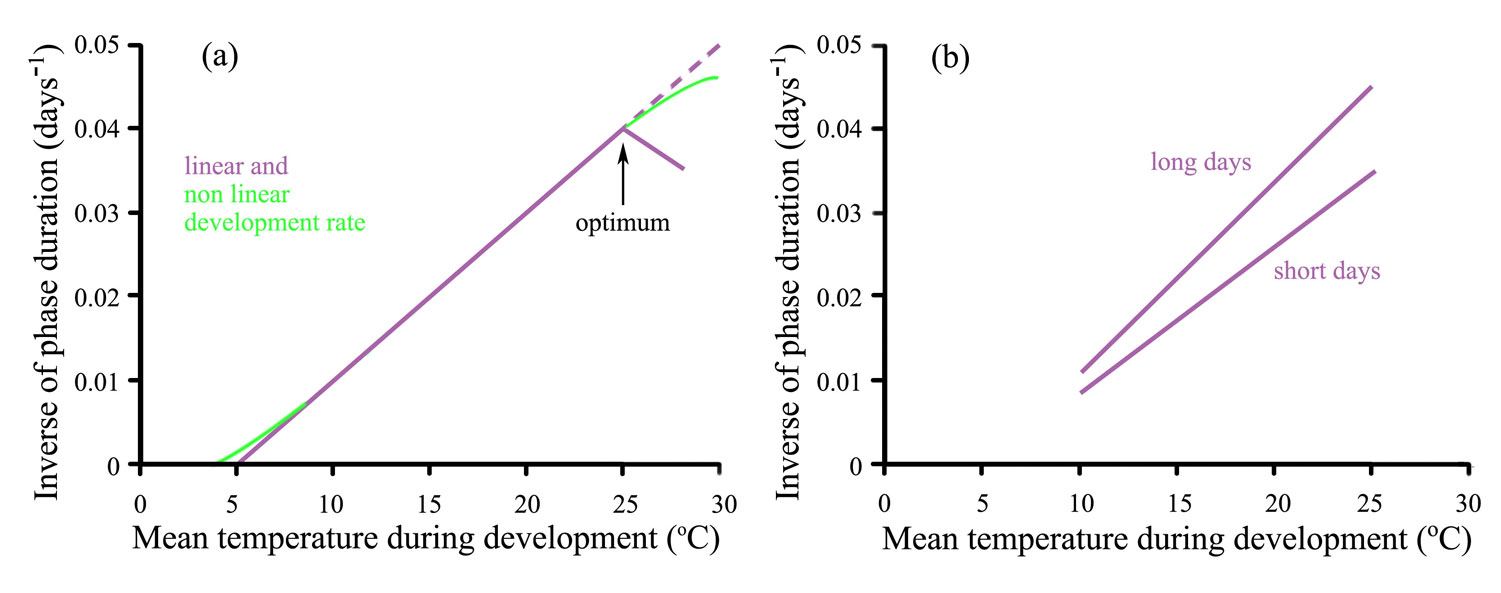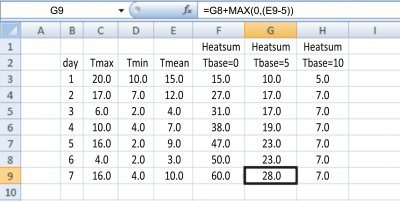# 14.2.2 - Thermal time and crop development

As already described, the time taken for plants and their organs to develop within their dynamic temperature range decreases as temperature increases. This was first quantified in the eighteenth century when the French physiologist Reaumur showed that grapes growing in different regions and seasons ripened when the accumulated daily temperature, ti , reached a (fairly) constant value, k, which is known as day degrees, heat units or heatsum. In equation (1) daily temperature is the mean of maximum and minimum temperatures, the subscript i refers to days and the summation was from winter until grape ripening. The units are °C.d.

$\displaystyle\sum\limits_{winter}^{ripening} t_i=k \tag{14.1}$

The system of accumulating temperature in this way is known as thermal time and can be applied to the life cycle of a plant or organ, or a phase of a plant’s development such as the seed-filling phase or the expansion of a single leaf.

One weakness of this system is that temperature accumulates above 0°C while in reality, for many species, development stops at temperatures well above freezing.  Instead of accumulating temperatures above 0°C, thermal time systems now use a base or threshold temperature, tbase, which varies with species and is estimated as the value that provides the most constant value of k. Using this system, heat sum is calculated from daily temperatures above tbase , as indicated by the subscript + in equation 2

$\displaystyle\sum\limits_{finish}^{start} (t_i-t_{base})_{+} =k \tag{14.2}$

The thermal time system can be simplified providing no daily temperature during a phase falls below tbase.  An example of the simplified system appears as a graph of duration of a phase, D, versus the mean temperature of the phase, ť (Figure 14.8a).  This line is defined by the duration of the phase, D, and the mean temperature of the whole phase above the base temperature, ť-tbase.  The product of these variables is the heatsum, k (equation 3).

$D(t-t_{base})=k \tag{14.3}$

## Fig14.8.jpgFigure 14.8. Duration of a phase in a plant’s life cycle, measured as time, decreases as mean temperature increases. For many plants, duration, when measured as thermal units, becomes a constant value. This thermal duration is called a heat sum, heat units or day degrees. It is calculated as the product of time duration and the mean temperature of the phase above a suitable base temperature. The base is 5°C in this graphical example that represents the temperature response of the phase from seedling emergence to flowering (anthesis) of a temperate plant such as wheat. The wheat developmental pattern is shown in (a) as a rectangular hyperbola (purple curve) in which the areas of the rectangles are equal. Expressing the same wheat data as the inverse of time duration against mean temperature (b) produces a straight line. The slope of the straight line is the inverse of the heat sum and the intercept on the x-axis is the base temperature.

Figure 14.8 shows the effect of temperature on phase duration from seedling emergence to flowering (anthesis). The curve in Figure 14.8a is a rectangular hyperbola, meaning that the areas of rectangles defined by the graph are constant, so in the examples $D_1(t_1'-t_{base})=D_2(t_2'-t_{base})$. For example a duration of 100 days at a mean temperature of 10°C represents a heat sum of 500 °C.d above a base temperature of 5°C i.e. 100(10-5) = 500 °C.d.  The relationship can be linearised by plotting the inverse of duration 1/D against mean temperature (Figure 14.8b). 1/D is defined as the mean rate of development, with units of day-1, and is equivalent to the proportion of development per day (equation 4).

$\frac{1}{D}=\frac{1}{k}(t'-t_{base})_{+} \tag{14.4}$

Extrapolation of the line to the x-axis provides an estimate of tbase and the slope of the line, 1/k, is the heatsum.  The advantage of plotting results in this way is that it provides a test of whether the thermal time system works for the data.

According to this example for wheat, a 2°C warming above a mean of 15°C would reduce the number of days to anthesis from 50 to ~42(500 °C.d / (15°C - 5°C) = 50 days and 500 °C.d / (17°C - 5°C) = 41.7 days).  Reduced duration can mean less crop growth because of fewer days for intercepting solar radiation and photosynthesis.

Rate of development is not always linearly related to mean temperature (Figure 14.9a).  At high temperatures the development rate of some plant phases decreases, showing a clear optimum temperature (arrowed).  This cardinal temperature along with the base temperature, defines the boundary of the dynamic temperature range for the phase, organ or species.  An optimum temperature is more likely to appear when plants are exposed to high temperature when light levels are relatively low.  Rate of development is affected by both temperature and photoperiod in photoperiod-sensitive species. So while development responses remain linearly related to temperature the slopes change with photoperiod; a long-day plant develops more rapidly in long days than in short days (Figure 14.9b).

## Fig14.9.jpgFigure. 14.9. Exceptions to the simple thermal time system shown in Figure 14.8. At temperatures close to the base temperature and at high temperatures development may diverge from a linear response of development rate to temperature (shown in (a) as the green departures from the purple straight line). In some species and phases there is a reduced development rate with higher temperature, shown in (a) by the purple line declining beyond the optimum). A long-day plant shows more rapid development in long days than short days over a wide range of temperatures (b).  Short-day plants show the reverse response. Based on Angus et al. (1981).

Thermal time can be used to predict crop development and the stages when herbicides, insecticides and fertiliser should be applied.  This is useful for farmers who manage crops over distances of hundreds of kilometres and need to visit them at a particular stage of development.  Thermal time can also be used to back-calculate the best sowing date to minimise the risk of frost or excessive heat damage at sensitive stages of development.

## Fig14.10.jpgFigure 14.10. Spreadsheet to calculate heatsum from daily maximum and minimum temperatures and a base temperature. Daily mean temperatures below the base do not contribute to heatsum, as shown by the equation in cell G9.

The dynamic ranges of temperatures for subtropical and tropical species are much higher than for this example of temperate wheat.  Consequently the base temperature will be higher and the heatsum different.  Nevertheless the method for calculating thermal time is similar.

Practical Notes: It is easy to calculate heatsums from temperature data that you measure yourself or download from the internet. Figure 14.10 is a spreadsheet showing a week’s accumulation of heatsums using different base temperatures. When collecting temperature data, remember to shade the thermometer, allow free air circulation and install it at the standard height for your region (1.2 m above ground in Australia).  To test the relationship between heatsum and phasic development you can record development of a phase of plant development in several environments by growing plants at different times of year or in different locations. For that, you need to record on your temperature spreadsheet the date of the start of the chosen phase (maybe the sowing date) and the date of the end of the phase (maybe flowering). Work out the thermal time for each day of the phase like in Figure 14.10, and add all sums together to produce the heatsum for the phase. Compare that result with data you collect from other planting dates. Are the heatsums the same despite different temperatures? If you calculate the mean temperature for the phase on your spreadsheet and the number of days from start to finish of the phase you can graph your combined data like in Figure 14.8. If you have enough sowing dates you will be able to work out the real base temperature for that phase of the chosen species.

### Some Definitions:

Development refers to the changes in form or ontogeny of plants (and animals) rather than growth, which means the increase of mass.  While normally growth and development are loosely related, it is possible to have growth without development, for example the continuous growth of vegetative buffalo grass, or to have development uncoupled from growth, for example when the flowering date of stunted drought-affected wheat is similar to the flowering date of well-watered wheat.

Phasic development relates to phases in a plant’s life cycle.  For example the phases in the life cycle of an annual crop are vegetative (between seedling emergence and floral initiation), reproductive (between floral initiation and flowering, called anthesis in wheat) and grain filling (between anthesis and physiological maturity)

Phenology is the science of the influence of climate on the occurrence of biological events